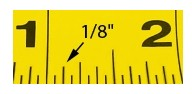# Can You Show Me A Inch From A Ruler?

This question is somewhat cryptic with many ways in which the question can be interpreted.

A ruler comes in two forms; metric and English.  A metric ruler is easy to read and deal with centimetres and millimetres only, there is no need to worry about fractions.  The larger lines which have numbers next to them on these rulers are centimetres and the very small lines are millimetres.

The way in which to read a metric ruler is by placing it next to or on the item which is to be measured then read the numbers and the small lines.

If for example you are measuring an item which is 17 large lines and 4 small lines, then this can be read as either 17.4 centimetres or 174 millimetres as there are 10 millimetres (or 10 small lines) in 1 centimetre.

English rulers can be a bit more difficult to read as they deal with inches and fractions. As with the metric rulers, these have large lines with numbers next to them, but the spaces between the numbers are larger as they measure 1 inch.

There are two types of English rulers.  One measures in 16ths and the other measures in 8ths.

You can tell which is which by counting the smaller lines which are between the large ones.  The line in the middle of the larger lines is usually a bit longer than the smaller ones and these measure one half of an inch.

A typical English ruler is 12 inches long.
thanked the writer.